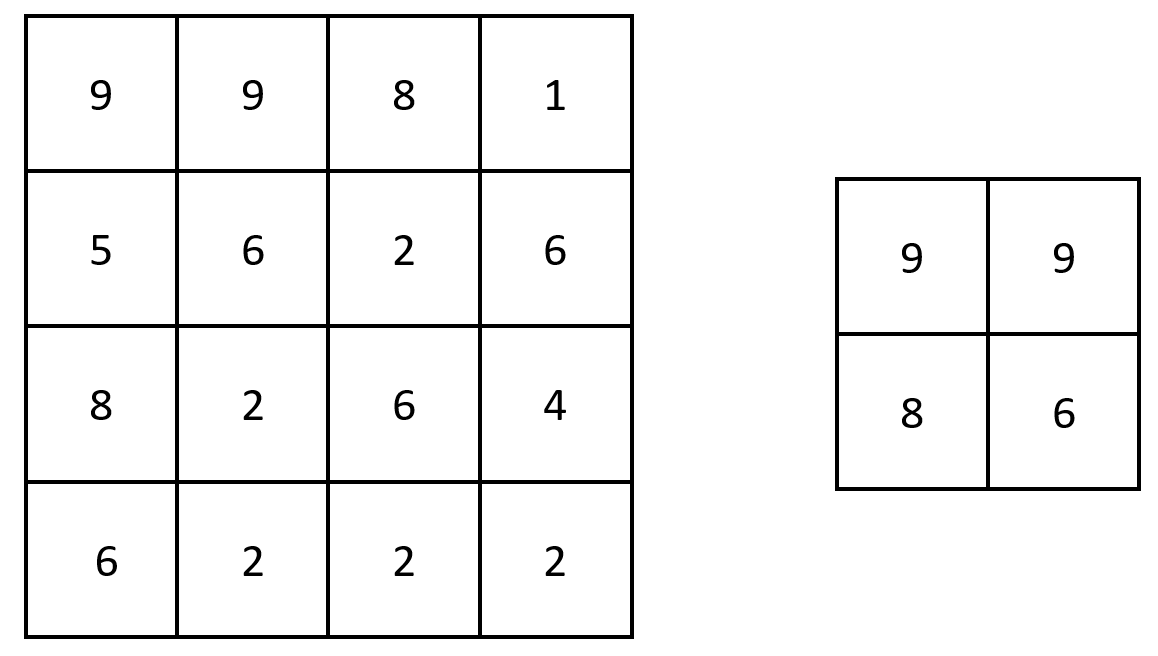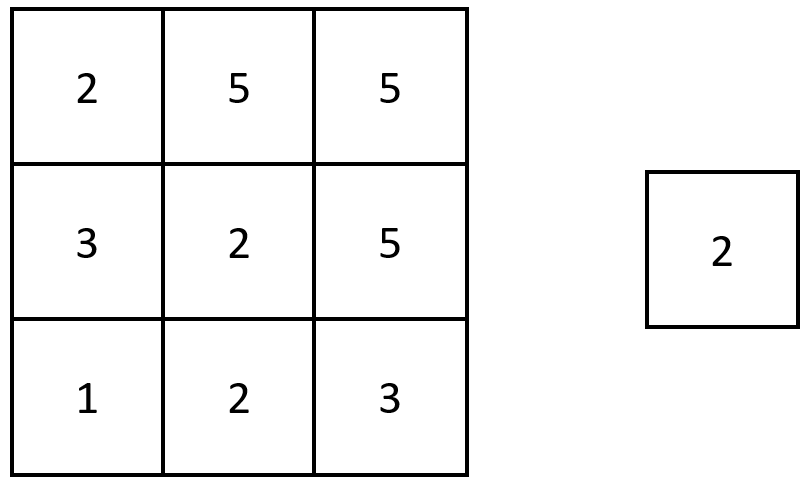2373. Largest Local Values in a Matrix

#### QUESTION:

You are given an n x n integer matrix grid.

Generate an integer matrix maxLocal of size (n - 2) x (n - 2) such that:

maxLocal[i][j] is equal to the largest value of the 3 x 3 matrix in grid centered around row i + 1 and column j + 1. In other words, we want to find the largest value in every contiguous 3 x 3 matrix in grid.

Return the generated matrix.

Example 1:``````Input: grid = [[9,9,8,1],[5,6,2,6],[8,2,6,4],[6,2,2,2]]
Output: [[9,9],[8,6]]
Explanation: The diagram above shows the original matrix and the generated matrix.
Notice that each value in the generated matrix corresponds to the largest value of a contiguous 3 x 3 matrix in grid.
``````

Example 2:``````Input: grid = [[1,1,1,1,1],[1,1,1,1,1],[1,1,2,1,1],[1,1,1,1,1],[1,1,1,1,1]]
Output: [[2,2,2],[2,2,2],[2,2,2]]
Explanation: Notice that the 2 is contained within every contiguous 3 x 3 matrix in grid.
``````

Constraints:

``````n == grid.length == grid[i].length
3 <= n <= 100
1 <= grid[i][j] <= 100
``````

#### SOLUTION:

``````class Solution {
func largestLocal(_ grid: [[Int]]) -> [[Int]] {
var result:[[Int]] = []
for indexI in 1...grid.count - 2 {
var arr:[Int] = []
for indexJ in 1...grid[indexI].count - 2 {
arr.append(largestLocalHelper(grid, indexI, indexJ))
}
result.append(arr)
}
return result
}

func largestLocalHelper(_ grid: [[Int]], _ i: Int, _ j: Int) -> Int {
return max(grid[i][j], grid[i-1][j-1], grid[i+1][j+1], grid[i-1][j],grid[i+1][j],grid[i][j-1],grid[i][j+1],grid[i+1][j-1],grid[i-1][j+1])
}
}
``````